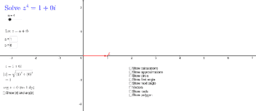# Complex Numbers as Vectors

Exploring the relationships between complex numbers and vectors.
•### 01 Vector Basics

•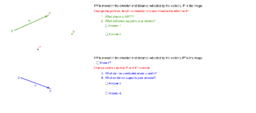### 02 Equal Vectors

•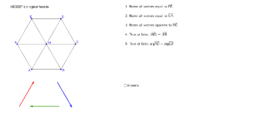### 03 Equal Vectors Exercises

••### 05 Subtracting Vectors

•### 06 Addition and Subtraction Exercises

•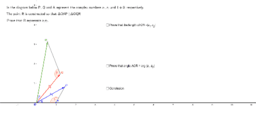### 07 Multiplying Complex Numbers

•### 08 Roots of Unity

•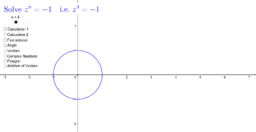### 09 Roots of Negative Unity

•